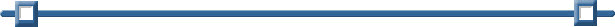Graphing Linear Inequalities This calculator has four shading patterns (controlled through "Attributes"). It rotates through the patterns as you graph inequalities.
 Example 1:    Graph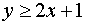Press. Continue pressing "clear" until the f(x) entry is gone. Enter. ( Press CTRL > to get the "greater than or equal to" symbol.) Notice that the line is solid. .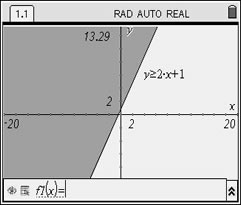To control the shading "color", arrow to the left of the inequality equation in the command line to the Attribute symbol.Arrow right and left to change the degree of the shade.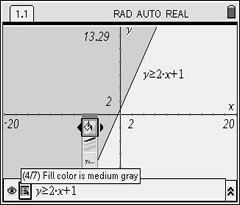Example 2:    Graph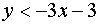Press. Continue pressing "clear" until the f(x) entry is gone. Enter. Notice that the line is dotted.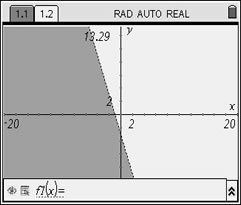Example 3:   Solve the system: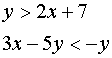You will need to isolate the y variable in the second equation so that it can be entered into the calculator. Solving algebraically, (be careful of the direction of the inequality symbol in this problem.)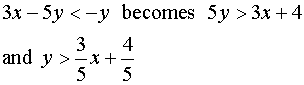The answer is the double shaded region on the graph.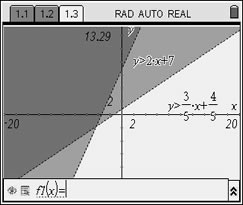Example 4:  Solve: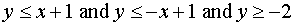Enter all three equations. Answer is the overlapped shading.